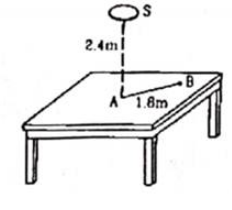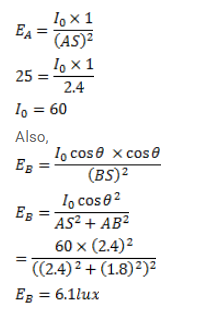# Figure shows a small diffused plane source SQuestion:

Figure shows a small diffused plane source $S$ placed over a horizontal table- top at a distance of $2.4 \mathrm{~m}$ with its plane parallel to the table-top. The illuminance at the point A directly below the source is 25 lux. Find the illuminance at a point $B$ of the table at a distance of $1.8 \mathrm{~m}$ from A.Solution: